## Questions

1.
1. What is meant by the following terms:
2. isotope.
2. The table shows how the activity (disintegrations per minute) of a sample of carbon-14 varies with time (in years).
 Time (grs) 0 2500 5000 7500 10000 12500 17250 20000 Disintegration/min 15 11 8 5 4 3.2 1.6 1.2
1. Plot a graph of activity against time (x-axis).
2. Estimate the half-life of carbon-14 from the graph.
3.
1. Draw a labeled diagram of a Geiger- Muller tube.
2. Explain how it detects radioactive particles/rays.
4. State one use of radioactivity in each of the following;
1. Medicine
2. Agriculture.
3. Radon gas 222 86Rn decays by emission of α particles. Show by use of an equation the transformation of the gas.
4. Give two uses of cobalt - 60 as a Radioactive source.
2. One of the isotopes of Uranium has a half life of 576 hours.
1. Complete the table to show how the mass varies with time from an initial mass of 1280 mg.
 Time (Hours) 576 1152 1728 2304 Mass (mg)
2. Explain whether the mass of the isotope will eventually reduce to zero.
3. State two factors that determine the extent of the damage to the body cell caused by the radiation from radioactive substances.
4. How many neutrons does the nuclide 235 92U contain?
5. Name the quantities, which must be measured so as to determine the half-life of a radioactive sample whose half-life is known to be a few hours.
6. Explain why α particles are more ionizing than β particles.
7. A radioactive carbon -14 decays to Nitrogen by beta emissions as shown.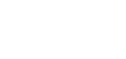Determine the values of x and y in the equation.
8. Alpha particles are more ionizing than Beta particles. Give one reason for this.
9. In a sample there are 5.12 x 1020 atoms of Krypton 92 initially. If the half-life of Krypton is 3.0s, determine the number of atoms that will have decayed after 6s
10. Cobalt 60 is a radioisotope that has a half-life of 5.25 years. What fraction of the original atoms in a sample will remain after 21 years.
11. A nucleus is represented by 107 32x
State the number of neutrons in a nucleus.
12.
1. The figure below shows the path of radiation from a radioactive source after entering a magnetic field. The magnetic field is directed into the paper and is perpendicular to the place of the paper shown in the figure below.2. Below is a nuclear reaction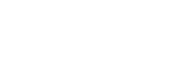2. Determine the values of X and Y.
3. The figure below shows a device for producing metal foils of constant thickness. Any change in the thickness can be detected by the Geiger tube and recorded by the Geiger cooler. The pressure exerted by the roller is then adjusted to keep the thickness constant.1. State the change in the metal foil that will lead to a decrease in the Geiger counter reading.
3. State the change in the roller pressure that should be made as a result of this decrease in the Geiger counter reading.
5. Explain why a source emitting (alpha) particles only would not be suitable for this device.
6. Explain why a radioactive source of a half-life of 1,600 years is more suitable for use in this device than one of half-life of 8 minutes.
13. The following is part of a radioactive decay series.Determine the values of a and b.
14.
1. A nucleus of an element X of atomic mass 238 and atomic number 92 decays by emitting 8 alpha particles and 10 beta particles and finally forms a nucleus of an element y. Write the equation of the reaction.
2. Each of the following sentences describes a particular radiation from different radioactive source. In each case name the type of radiation described and give a reason to support your choice.
1. The radiation is not affected by either a magnetic or electrostatic field.
Reason..........................
2. The radiation is emitted from 238 - U when it decays to 234 90
Reason..........................
3. The radiation is very strongly deflected by a weak magnetic field.
Reason..........................
15. The half-life of a certain radioactive substance is 57 days. Explain the meaning of this statement.
16. The figure below shows a Geiger Muller (G.M.) tube.1. Give the reason why the mica window is made thin.
2. Explain how the radiation entering the tube through the window is detected by the tube.
3. What is the purpose of the halogen vapour?
17. Balance the nuclear reaction equation below.18. Given that 5g of cobalt-60 is kept in a laboratory and it has a half-life of 5 years.
Calculate its mass after 15 years.

1.
1.
1. Radioactive Decay – spontaneous disintegration of the molecules and atom or emission of or α or β accompanied by release of energy and readjustment of the nuclide.
2. Isotope: Is a nuclide, which has same no. of proton but different atomic mass, same atomic number but different mass number, same chemical behavior but different properties.
2.
3.
1.2. -When an alpha or beta particle or gamma ray enters the tube through the mica window, they ionize the gas inside the tube.
-The electrons produced are accelerated by the central electrode and in turn they produce more electrons by collision.
-This avalanche of electrons forms a pulse of current, which, after amplification, is used to operate a rate meter or counter.
-If a small loud speaker is connected a click will be heard every time a particle or gamma photon enters the tube.
4.
1. Medicine: Deep penetrating gamma rays are used in the treatment of cancerous growth.
2. Agriculture: To determine the age of plant material and the movement of minerals implants.
2.
1.
 Time (Hours) 576 1152 1728 2304 Mass (mg) 640 320 160 80
2. It will be zero
3. -Dosage
-Penetrating power
-Part of body exposed
4. 235 – 92 = 143 Neutrons
5. -Mass of that radioactive material
-Time taken to reduce to half
6. α Particles doubly charged and attracted electrons more.
α More massive i.e more momentum and not easily deflected

7.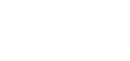14 = x + 0 thus x = 14
6 = 7 + y thus y = -1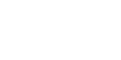8. α - particles doubly charged and attract electrons more.
α more massive i/e more momentum and not easily deflected.
9. After 3s number decayed
½ x 5.12 x 1020 = 2. 56 x 1020
Next 3s number decayed
= ½ x 5.12 x 1020 = 2.56 x 1020
∴ Total number decayed
= (1.28 + 2.56) x 1020
= 3.84 x 1020
10. No of T½ = 4
Thus fraction = (1/2)4 = 1/16
11. Number of neutrons = 107 – 32 = 65
12.
1.
1. Beta particle (β – particle)
2. A force is to the center of the circle implying negatively charged (Fleming’s L HR (etc)
2.
1. Alpha particle ( particle)
2. X- 88
Y – 228
3.
1. Increase in thickness
2. Increase in thickness reduces the number of radiations reaching the Geiger tube.
3. Increase roller pressure
4. Increase in roller pressure squeezes/ presses/ compresses metal sheet more reducing thickness of the foil coming out of them.
5. Alpha particles have low penetration power and so will not pass/ very few will pass though sheet.
6. If a half life is short, decay rate reduces very fast with time and so this sudden change may be mistaken a shaving resulted from increase in thickness OR would be detecting as change in thickness of foil, so longer half- life is more suitable
13. a= 234
b= 82
14.
1.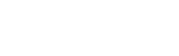2.
1. Gamma rays- not charges
2. Alpha particles: mass number decreases by 4 and atomic number decreases by 2
3. Beta particles: easily deflected because they are light and carries little charge.
15. The substance takes 57 days to reduce its remaining mass by half.
16.
1. Alpha particles are weak in penetrating solid. So it has to be thin to allow enough alpha particles to enter for ionization to take place.
2. It causes ionization of the gas inside. The +ve ions move to the –ve plate and –ve ions move to the +ve terminal. This causes a flow of charge which is amplified and then fed to the counter.
3. It controls the extent of ionization without causing avalanche flow of charge.
17.18. Year          Amount
0                    5
5                   2.5
10                 1.25
15                 0.625
The mass will be 0.625g

• ✔ To read offline at any time.
• ✔ To Print at your convenience
• ✔ Share Easily with Friends / Students